## Geometry: Finding Unknown Angles (Advanced Problems)

#### Problem 1: In the figure below, ABC and DEF are triangles. AC = AB, AB // DF, BC // ED, AC // EF and ∠ CAB = 70°. Find ∠ x, ∠ y and ∠ z.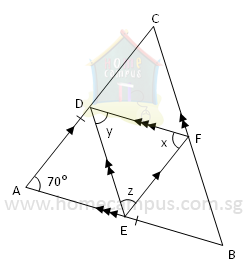Δ ABC is an isosceles triangle.
∠ ACB  =  ∠ ABC

=   (180°  −  70°)  ÷  2

=   55°
DF // AE and AD // EF
So, AEFD is a parallelogram.
∠ DAE  =  ∠ DFE

=   70°

∠ x  =  70°
DF // EB and DE // FB
So, DEBF is a parallelogram.
∠ EBF  =  ∠ EDF

=   55°

∠ y  =  55°
DE // CF and DC // EF
So, DEFC is a parallelogram.
∠ DCF  =  ∠ DEF

=   55°

∠ z  =  55°

#### Problem 2: Sally makes a flag using two triangles, ABC and CDE, on a straight pole XY as shown below. Triangle ABC is an equilateral triangle. Triangle CDE is a right-angled isosceles triangle with EC = ED and ∠ CED = 90°. Find the unknown angle, ∠ p, between the two triangles.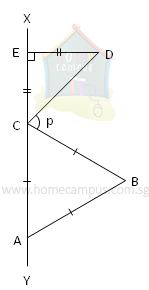Δ CDE is a right-angled isosceles triangle.
∠ ECD  =  ∠ EDC

=   (180°  −  90°)  ÷  2

=   45°
Δ ABC is an equilateral triangle.
∠ ACB = 60°
ECA is a straight line.

∠ ECD  +  ∠ p  +  ∠ ACB  =  180°

∠ p  =  180°  −  ∠ ECD  −  ∠ ACB

=   180°  −  45°  −  60°

=   75°

#### Problem 3: The figure below shows a parallelogram AECF inside another parallelogram ABCD. AB = AC, ∠ AEC = 53° and ∠ FDC = 38°. Find ∠ t.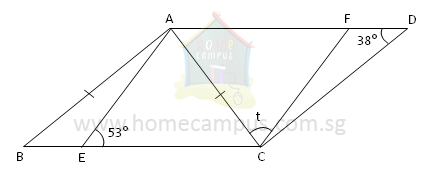Opposite angles of a parallelogram are equal.
In the Parallelogram ABCD,

=   ∠ FDC

=   38°
Δ ABC is an isosceles triangle as AB  =  AC.
In the Isosceles Triangle ABC,
∠ ABC  =  ∠ ACB  =  38°
Sum of adjacent angles of a parallelogram is 180°.
In the Parallelogram AECF,

∠ EAF  =  180°  −  ∠ AEC

=   180°   53°

=   127°
Opposite angles of a parallelogram are equal.
∠ FCE  =  ∠ EAF

=   127°
∠ t  =  ∠ FCE  −  ∠ ACE

=   ∠ FCE  −  ∠ ACB

=   127°  −  38°

=   89°

#### Problem 4: In the figure below, ABCD is a rhombus and ADE is a straight line. ∠ DAB = 51° and ∠ DCE = 42°. Find ∠ x and ∠ y.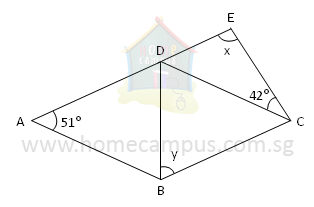Opposite angles of a rhombus are equal.
In the Rhombus ABCD,

∠ BCD  =  ∠ DAB  =  51°
Hence, DE // BC and BCED is a trapezium.
In the Trapezium BCED,

∠ x  =  180°  −  ∠ BCE

=   180°  −  ∠ BCD  −  ∠ DCE

=   180°  −  51°  −  42°

=   87°
BC = CD as all sides of a rhombus are equal.
Triangle BCD is an isosceles triangle.

∠ y  =  ∠ BDC

=   (180°  −  51°)  ÷  2

=   64.5°

#### Problem 5: ABC is a right-angled triangle with ∠ BAC = 90°. OA = OD = OF. EO // CA and DO // BC. Find ∠ x.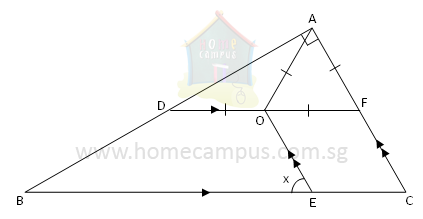Triangle AOF is an equilateral triangle.

∠ AOF  =  ∠ OFA  =  ∠ FAO  =  60°
DAF = 90°
=   30°
Triangle AOD is an isosceles triangle.

∠ OAD  =  ∠ ODA  =  30°

∠ ODB  =  180°  −  ∠ ODA  =  150°
DBEO is a trapezium.

∠ DBE  =  180°  −  ∠ ODB

∠ DBE  =  180°  −  150°  =  30°
ABC = DBE = 30°
In Triangle ABC,

∠ ACB  =  180°  −  ∠ BAC  −  ∠ ABC

=   180°  −  90°  −  30°

=   60°
FCE = ACB = 60°
OECF is a trapezium.

∠ CEO  =  180°  −  ∠ FCE

=   120°
BEC is a straight line.

∠ x  =  180°  −  ∠ CEO

=   180°  −  120°

=   60°